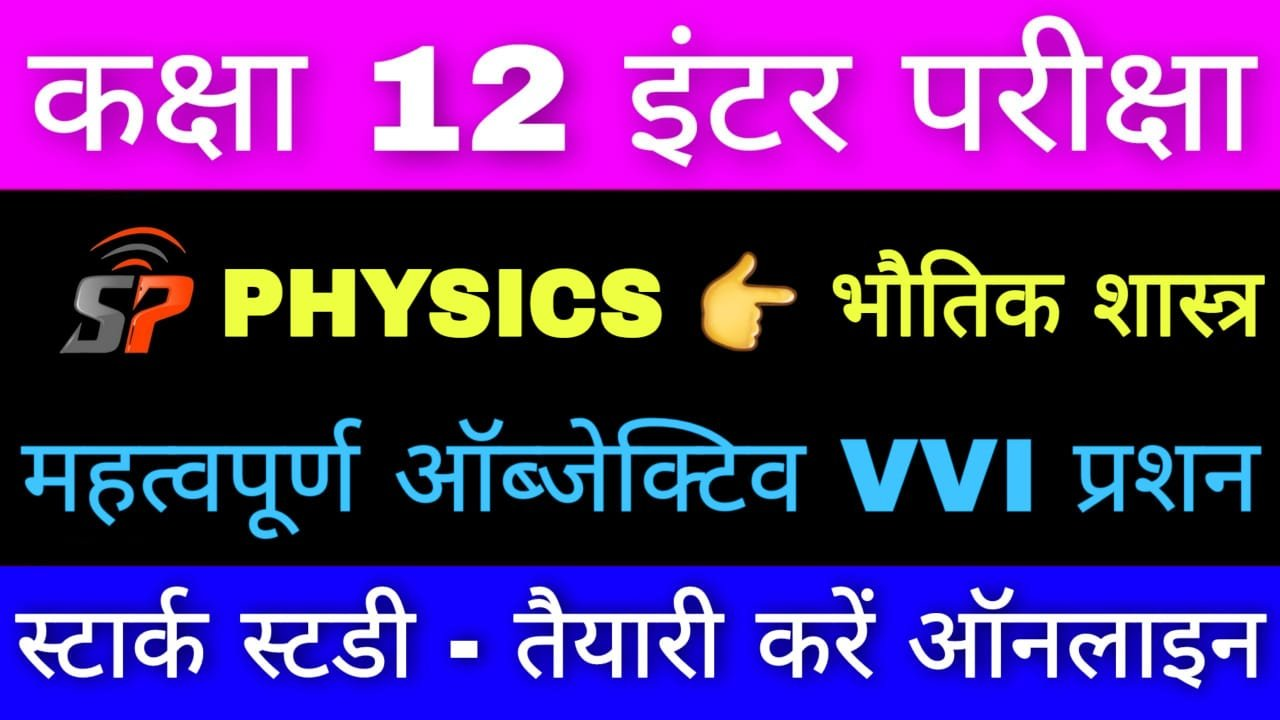Class 12th Physics Chapter 6 Electromagnetic Induction VVI Objective Question

ELECTROMAGNETIC INDUCTION

1. Unit of self-induction is

(A) Weber Title

(B) Ohm

(C) Henry

(D) Gauss

2. The direction of eddy current is obtained using

(A) Kirchhoff’s laws

(B) Lenz’s law

(C) Planck’s law

(D) none of these

3. Lenz’s law is associated with

(A) charge

(B) mass

(C) energy

(D) principle of conservation of momentum

4. The working of dynamo is based on the principle of

(A) heating effect of current

(B) electromagnetic induction

(C) magnetic induction

(D) electric induction

5. Eddy current can provide

(A) retardation in speed

(B) heat

(C) separation of metals from non-metals

(D) all of these

6. The S.I. unit of flux of magnetic field

(A) tesla

(B) henry

(C) weber

(D) joule-second

7. The law governing electromagnetic induction is called

(A) Newton’s law

(C) Ohm’s law

(D) Ampere’s law

8. Lenz’s law is a consequence of the law of conservation of

(A) charge

(B) energy

(C) mass

(D) momentum

9. “Volt is the unit of

(A) induced emf

(B) induced current

(C) potential difference

(D) both ‘A’ and ‘C’

10. The principle on which the working of dynamo is based is

(A) heating effect of current

(B) electromagnetic induction

(C) induced magnetism

(D) induced electricity

11. An inductor may store energy in its

(A) electric field

(B) coil

(C) magnetic field

(D) none of these

12. The working of an induction coil is based on the principle of

(A) electric induction

(B) magnetic induction

(C) electromagnetic induction

(D) none of these

13. An induction coil give

(A) a high voltage at high current

(B) a high voltage at low current

(C) a low voltage at high current

(D) a low voltage at low current

14. A dynamo

(A) creates mechanical energy

(B) creates electrical energy

(C) converts mechanical energy to electrical energy

(D) converts electrical energy to mechanical energy

15. An electric motor

(A) creates mechanical energy

(B) creates electrical energy

(C) converts mechanical energy to electrical energy

(D) converts electrical energy to mechanical energy

16. Voltage is induced where there is a relative motion between a conductor and

(A) another conductor

(B) a magnetic field

(C) an electrostatic field

(D) None of these

17. The e.m.f. produced in a wire by its motion across a magnetic field does not depend upon

(A) the length of the wire

(B) the diameter of the wire

(C) the composition of wire

(D) both ‘B’ and ‘C’

18. The e.m.f. induced in a conductor that is moved in a magnetic field depends on the

(A) initial value

(B) final value

(C) total charge

(D) rate of change of the magnetic flux thrown conductor

19. A magnet is dropped down a long vertical copper tube. The acceleration of the magnet will

(A) be zero after some time

(B) be the same through out

(C) increase to same value

(D) increase continuously

20. Two inductors each of inductance ‘L’ are joined in parallel. Their equivalent inductance is

(A) 2L

(B) L/2

(C) L

(D) zero

21. If an inductor having inductance L is joined to another identical inductor with its one end joined, the resultant inductance would become

(A) 2L

(B) L/2

(C) zero

(D) L/4

22. If 100% flux of coil of inductance L1 is linked with the coil of inductance L2 then coefficient of coupling is

(A) unity

(B) infinity

(C) zero

(D) none of these

23. The self inductance of a coil is 5 mH. If a current of 2 A is passed, the magnetic flux linked with the coil will be

(A) 1 Wb

(B) 0.1 Wb

(C) 0.01 Wb

(D) 0.001 Wb

24. If there are N, and N, turns in the primary and seconday coils respectively in a step-down transformer, then

(A) N1 >N

(B) N2>N1

(C) N1= N2

(D) N1= 0

25. Transformer works in case of

(A) only d.c.

(B) only a.c.

(C) both a.c. and d.c.

(D) none of these

26. The material most appropriate for core of a transformer is

(A) soft iron

(B) copper

(C) stainless steel

(D) alnico